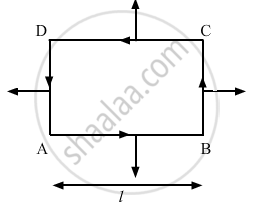Advertisement Remove all ads

# The Magnetic Field Existing in a Region is Given by → B = B 0 ( 1 + X 1 ) → K . a Square Loop of Edge L and - Physics

Sum

The magnetic field existing in a region is given by  vecB = B_0(1 + x/1)veck . A square loop of edge l and carrying a current i, is placed with its edges parallel to the xy axes. Find the magnitude of the net magnetic force experienced by the loop.

Advertisement Remove all ads

#### Solution

Given:
Magnetic field, vecB = B_0(1 + x/1)veck
Length of the edge of a square loop = l
Electric current flowing through it = i
As per the question, the loop is placed with its edges parallel to the XY axes.In the figure, arrow denotes the direction of force on different sides of the square.
The net magnetic force experienced by the loop,
vecF = ivecl  xx vecB
Force on AB:
Consider a small element of length dx at a distance x from the origin on line AB.
Force on this small element,
dF = iB_0 on the full length of AB,
FAB = $\int\limits_{x=0}^{x=0}$ iB_0 (1 + x/l)

= iB_0 $\int\limits_{x=0}^{x=0}$ (dx + 1/l xdx)
= iB_0(l + 1/2)

= (3iBgl)/(2)
Force on AB will be acting downwards.
Similarly, force on CD,
F_2 = iB_0 (l + l/2)

=(3iBgl)/(2)
Force on AB will be acting downwards.
Similarly, force on CD,
F_2 = iB_0 (l + 1/2)
= (3iBgl)/2
So, the net vertical force = F1  F2 = 0
Force on AD,
F_4 = iB_0l (1 + 1/l)
= 2iB0l
Force on BC
F_4 = iB_0l(1 + 1/l)
=2iB0l
So, the net horizontal force = F4F3 = iB0l

Is there an error in this question or solution?
Advertisement Remove all ads

#### APPEARS IN

HC Verma Class 11, Class 12 Concepts of Physics Vol. 2
Chapter 12 Magnetic Field
Q 27 | Page 232
Advertisement Remove all ads

#### Video TutorialsVIEW ALL 

Advertisement Remove all ads
Share
Notifications

View all notifications

Forgot password?